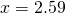# Solution assignment 07 Trigonometric equations

### Assignment 7

Solve the following equation for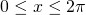: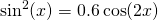### Solution

In the right-hand side we write for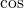: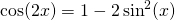We could also have applied other formulas but this one fits better to the left-hand side.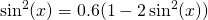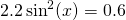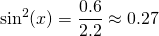The equation: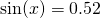has the following solution (within the domain):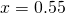or: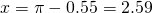The equation: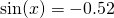has the solution: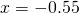(this one does not lie in the domain and is not valid)

or: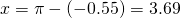Again, this solution does not lie in the domain and is not valid.

Thus the solutions are:or: